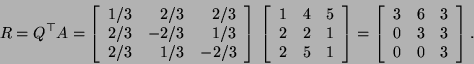MATH69.217* ANSWERS TO TEST 3 Fall 2001

1.
(a) State the definition of an orthonormal basis in. (b) Verify the identitywherestands for the dot product of vectorsand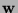in.

(a) An orthonormal basis inis a basis inconsisting of unit vectors perpendicular to each other. (b) We begin with the right hand side:Done.

2.
Find the entries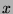,and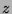in the following orthogonal matrix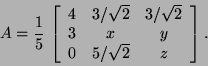From the fact that the first two columns are perpendicular, we obtain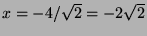. From the fact that the first and the last rows are perpendicular, we get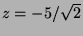. Finally, from the fact last two rows are perpendicular, we get.

3.
Find the projection of the vector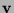onto the subspace spanned by the vectors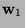and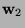, where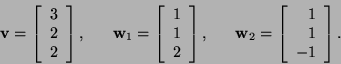Begin by checking that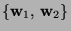is an orthogonal system.

First we check. Hence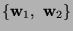is indeed and orthogonal system. The required projection is4.
Find the QR factorization of the matrix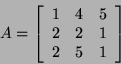Write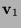,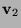and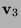for the columns of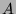. Put. Let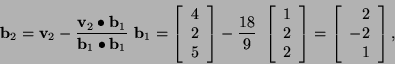and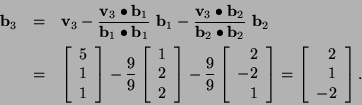Upon normalization, we get an ONB:The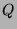matrix in the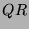-decomposition ofis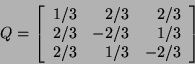and the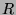matrix is